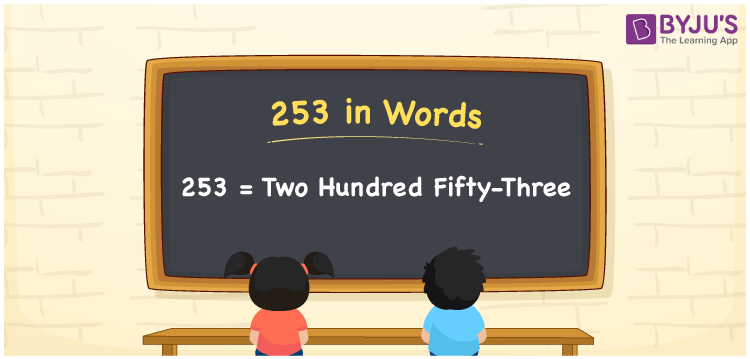# 253 in words

253 in words is written as Two Hundred and Fifty Three. 253 represents the count or value. The article on Counting Numbers can give you an idea about count or counting. The number 253 is a 3 digit number that is used in expressions related to money, days, distance, length, weight and so on. Let us consider an example for 253. “This paragraph has Two Hundred and Fifty Three words.”

 253 in words Two Hundred and Fifty Three Two Hundred and Fifty Three in Numbers 253

## 253 in English Words## How to Write 253 in Words?

We can convert 253 to words using a place value chart. The number 253 has 3 digits, so let’s make a chart that shows the place value up to 3 digits.

 Hundreds Tens Ones 2 5 3

Thus, we can write the expanded form as:

2 × Hundred + 5 × Ten + 3 × One

= 2 × 100 + 5 × 10 + 3 × 1

= 253

= Two Hundred and Fifty Three.

253 is the natural number that is succeeded by 252 and preceded by 254.

253 in words – Two Hundred and Fifty Three.

Is 253 an odd number? – Yes.

Is 253 an even number? – No.

Is 253 a perfect square number? – No.

Is 253 a perfect cube number? – No.

Is 253 a prime number? – No.

Is 253 a composite number? – Yes.

## Solved Example

1. Write the number 253 in expanded form

Solution: 2 × 100 + 5 × 10 + 3 × 1

We can write 253 = 200 + 50 + 3

= 2 × 100 + 5 × 10 + 3 × 1.

## Frequently Asked Questions on 253 in words

Q1

### How to write the number 253 in words?

253 in words is written as Two Hundred and Fifty Three.
Q2

### Is 253 divisible by 3?

No. 253 is not divisible by 3.
Q3

### Is 253 a prime number?

No. 253 is not a prime number.